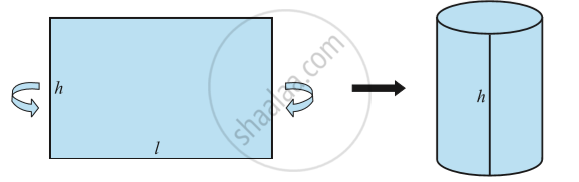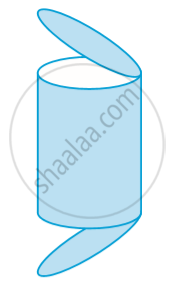Share

# Surface Area of a Right Circular Cylinder

#### notes

A cylinder is to be covered with coloured paper. The  length is just enough to go round the cylinder and whose breadth is equal to the height of the cylinder as shown in following fig.The area of the sheet gives us the curved surface area of the cylinder. Note that the length of the sheet is equal to the circumference of the circular base which is equal to 2πr.
So, curved surface area of the cylinder
= area of the rectangular sheet = length × breadth
= perimeter of the base of the cylinder × h
= 2πr × h

Therefore,
Curved Surface Area of a Cylinder = 2πrh

where r is the radius of the base of the cylinder and h is the height of the cylinder.

In the case of a cylinder, unless stated otherwise, ‘radius of a cylinder’ shall mean’ base radius of the cylinder’.

If the top and the bottom of the cylinder are also to be covered, then we need two circles (infact, circular regions) to do that, each of radius r, and thus having an area of πr2 each in following fig.The total surface area as 2πrh + 2πr2 = 2πr(r + h).
So,
Total Surface Area of a Cylinder = 2πr(r + h)

Remark:
The value of π is a non-terminating, non-repeating decimal. usually take its value as approximately equal to 22/7 or 3.14.

### Shaalaa.com

Surface Area of a Right Circular Cylinder [00:12:46]
S
0%

S